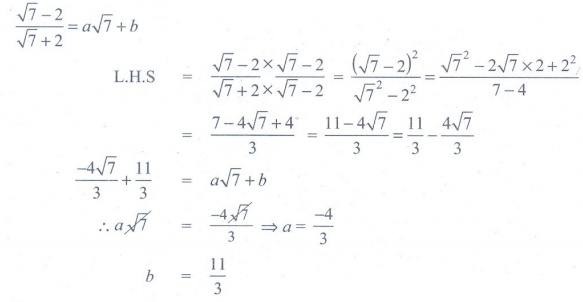Home | | Maths 9th std | Exercise 2.7: Rationalisation of Surds

# Exercise 2.7: Rationalisation of Surds

Maths : Real Numbers : Book Back, Exercise, Example Numerical Question with Answers, Solution : Exercise 2.7: Rationalisation of Surds

Exercise 2.7

1. Rationalise the denominator2. Rationalise the denominator and simplify3. Find the value of a and b if [√7 −2]  / [√7 +2] = a√7 +b4. If x = √5 + 2, then find the value of x2 + [1/x2]5. Given √2 = 1.414 , find the value of [8 -5√2] / [3 -2√2] (to 3 places of decimals).Tags : Numerical Problems with Answers, Solution | Real Numbers | Maths , 9th Maths : UNIT 2 : Real Numbers
Study Material, Lecturing Notes, Assignment, Reference, Wiki description explanation, brief detail
9th Maths : UNIT 2 : Real Numbers : Exercise 2.7: Rationalisation of Surds | Numerical Problems with Answers, Solution | Real Numbers | Maths